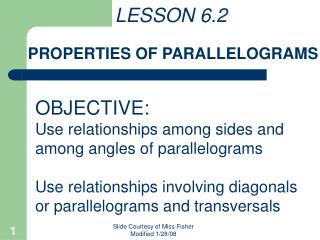DownloadDownload PresentationLESSON 6.2 PROPERTIES OF PARALLELOGRAMS

# LESSON 6.2 PROPERTIES OF PARALLELOGRAMS

Download Presentation## LESSON 6.2 PROPERTIES OF PARALLELOGRAMS

- - - - - - - - - - - - - - - - - - - - - - - - - - - E N D - - - - - - - - - - - - - - - - - - - - - - - - - - -
##### Presentation Transcript

1. LESSON 6.2 PROPERTIES OF PARALLELOGRAMS OBJECTIVE: Use relationships among sides and among angles of parallelograms Use relationships involving diagonals or parallelograms and transversals Slide Courtesy of Miss Fisher Modified 1/28/08

2. Definitions Check for Understanding: Starting with  K move counterclockwise around JKLM to name pairs of consecutive angles. Consecutive angles of a polygon ____________________ share a common side. In JKLM, J and M are consecutive angles, as are J and ___. J and ___ are ____________ angles. K L opposite Slide Courtesy of Miss Fisher Modified 1/28/08

3. Theorems Check for Understanding: If RT and US bisect one another at point M, name two pairs of  segments. Theorem 6.1 ___________ sides of a parallelogram are . Theorem 6.2 Opposite angles of a parallelogram are ____. Theorem 6.3 The diagonals of a parallelogram _________ each other. Opposite  bisect Slide Courtesy of Miss Fisher Modified 1/28/08

4. Theorems Con’t Theorem 6.4 If three (or more) parallel lines cut off congruent segments on one transversal, then _____________________________ _________________________________ _________ they cut off congruent segments on every transversal. BD  DF Slide Courtesy of Miss Fisher Modified 1/28/08

5. EXAMPLE #1 Find the value of x in ABCD. Then find mA. Opposite angles of a parallelogram are _________ so, mB = x + 15 = 60 + 15 congruent = 75 x + 15 = 135 - x 2x + 15 = 135 *mA + mB = 180 2x = 120 mA + 75 = 180 x = 60 mA = 105 *Recall, consecutive angles in a parallelogram are supplementary, since __________________________. // lines  same-side int. s supp. Slide Courtesy of Miss Fisher Modified 1/28/08

6. EXAMPLE #2 Find the values of x and y in KLMN. Diagonals of a parallelogram ______ each other, so bisect Substitute 7y -16 for x in the second equation. x = 7y – 16 and 2x + 5 = 5y x = 7(3) – 16 2(7y–16) + 5 = 5y x = 21 – 16 14y – 32 + 5 = 5y x = 5 14y – 27 = 5y -27 = -9y Substitute 3 for y in the first equation. 3 = y Slide Courtesy of Miss Fisher Modified 1/28/08

7. EXAMPLE #3 In the figure DH || CG || BF || AE, Find EH. If three (or more) parallel lines cut off congruent segments on one transversal, then __________________________________________________________________ they cut off congruent segments on every transversal, so EF = FG = GH. EH = EF + FG + GH EH = 2.5 + 2.5 + 2.5 EH = 7.5 units Slide Courtesy of Miss Fisher Modified 1/28/08

8. Assignment: pg 297 #2-16, even, 17, 19, 22, 34-35, 39-41 Slide Courtesy of Miss Fisher Modified 1/28/08